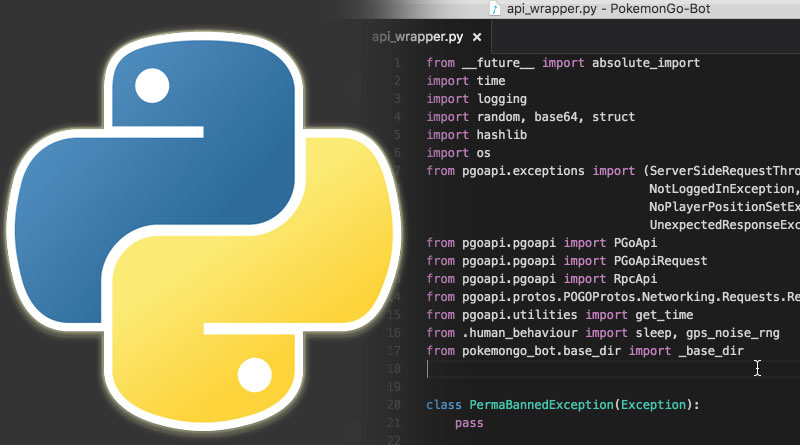# Some Basic Tic-Tac with PythonThe article is about Python language where I have focused on any project or a program which is coded in Python language.

It is necessary for us to understand that if we are going to implement server less Python program the first thing we need to resolve is challenges which we all are facing collectively. For example, building a programmers and coders community.

This is all together efforts of for inviting the various coders to one platform which is again server less and helping them to face their real-time challenges.

I am directly jumping on the key areas where these are the most commonly used function and they are mentioned as under:

### 1. Lambda Function:

The biggest advantage of using Lambda is all about using this function which has two arguments. If you are looking for some sequence segments it will the fresh element.

Knowing more about the map() function

With the best flexibility with Lambda function you don’t need to take the mess of defining the name of the function for example function named as abc(), and xyz().

The result will get showcased as under:

```>> xyz = [22.2, 30.5, 32.3, 35.8]
>>> abc = map (lambda a: (float(7)/5)*x + 30,xyz)
>>> print abc
[102.XX, 97.700XXX]
>>> b = map(lambda x: (float(5)/9)*(x-32), Fahrenheit)
>>> print C
[39.2000000000XXXX3, 36.5, 37.3000000000XXXX4, 37.799999999XXXXX7]
>>
```

Hence, map() is declared and worked as a universal function and can be applied everywhere in the list.

### 2. Implementing Wire Logic in Python:

There are many ways to implement wire logic when we talk about Python, however, With the help of OOPS concepts we can definitely create behavioral logical circuits. However, we will be using the gates which are so common for any study of circuit analysis these gates are namely, (AND, XOR, OR, NAND).

Some examples of Python Classes

```class Connector :
def __init__(You, Title, Name, abc=0, xyz=0):
self.value = You
self.owner = Title
self.name  = Name
self.xyz  = xyz
self.connects = []
self.abc = abc```

Focusing output

```def connect(self, inputs):
if not isinstance(inputs, list):
inputs = [inputs]
for input in inputs:
self.connects.append(input)```

What this program will print as an output

```def set(self, value):
if self.value == value:
return
self.value = value
if self.activates:
self.title.evaluate()
if self.monitor:
print("Connector {0}-{1} set to {2}".format(self. title.name,
self.name, self.value))
for con in self.connects:
con.set(value)```

### 3. Using web plugin in Python:

Step 1: Using a View: we all understand that whenever the user enters any queries and the browser makes a connection there is a process called request and respond.

What makes Python a special way of a programming language is that Python needs to be programmed between the server and the server.

Step 2: Building Common Gateway: You might be aware of “CGI” most commonly used in web server. This is about writing few lines code in Python and it was accepted widely. However, after using “WSGI”.

How you can use CGI with Python

Sample Code:

```import cgi
import cgitb; cgitb.enable()  # for troubleshooting
print "Content-type: text/html"
print
print """

<html>
<body>
<h3> Sample CGI Script </h3>
"""
form = cgi.FieldStorage()
message = form.getvalue("message", "(no message)")
print """
<p>Previous message: %s</p>
<p>form
<form method="post" action="index.cgi">
<p>message: <input type="text" name="message"/></p>
</form>
</body>
</html>
""" % cgi.escape(message)```

Conclusion:

Going deep down in Python is great and as you explore it you face some challenges and you often rely on a plugin. My main motto is to exchange these challenges in various Python community and get collective solutions for all.